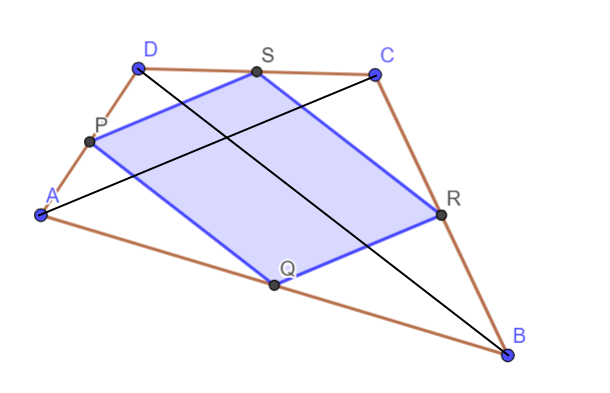#### You may also like### Polycircles

Show that for any triangle it is always possible to construct 3 touching circles with centres at the vertices. Is it possible to construct touching circles centred at the vertices of any polygon?### DOTS Division

Take any pair of two digit numbers x=ab and y=cd where, without loss of generality, ab > cd . Form two 4 digit numbers r=abcd and s=cdab and calculate: {r^2 - s^2} /{x^2 - y^2}.### Loopy

Investigate sequences given by $a_n = \frac{1+a_{n-1}}{a_{n-2}}$ for different choices of the first two terms. Make a conjecture about the behaviour of these sequences. Can you prove your conjecture?

### Why do this problem?

This problem introduces students to a surprising result which holds true for all quadrilaterals, although in the problem we invite them to explore convex quadrilaterals first. The GeoGebra interactivity provides a hook to engage students, and we hope the result will be intriguing to students, encouraging their curiosity and giving them a desire to persevere until they have proved the result.

### Possible approach

You may wish to begin by inviting students to draw their own quadrilaterals on dotty paper, which you can print off from our Printable Resources page. Once they have generated a few examples, invite them to explain anything they have noticed. This is a really good opportunity for discussion about the examples they chose - did their original quadrilaterals have any particular symmetry properties that might have caused the results they are finding? Can they find an example that doesn't work?

At this point, you might like to encourage students to explore using dynamic geometry, either by using the interactivity in the problem, the interactivity created by Alison Kiddle, or constructing their own using software such as GeoGebra, which is free to use.

Diagrams such as this one might be useful for helping students to construct a proof:As well as considering the shape formed, students could also calculate the area of the original quadrilateral and the new one formed by the midpoints, and look for a relationship.

### Key questions

What is special about the new quadrilateral formed by joining the midpoints?
Does it always happen?
Does it help to draw in the diagonals?
Are there any congruent shapes in your picture?
Does that help you to work out the relationship between the areas?

### Possible support

Students could start by exploring what happens when you join the midpoints of a triangle.